Equation - examples - page 54

1. Chewing gums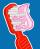For 3 chewing gums, you will pay 20 CZK less than 7 chewing gums. How much is 1 chewing gum and how much does a 5pcs package cost?
2. Mysterious number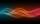The magician thinks the number: "The mysterious number is first divided by minus five, dividing the result by three, multiplying the number by ten, and dividing the resulting number by minus four. This gives result 5. Can you reveal the mysterious number?
3. The tubIf we drop the 5 l from the first tap and the from second 2 liters of water, the water in the tub will have a temperature of 25°C. If we drop 3 l from the first tap and from second 4 l of water, the water in the tub will have a temperature of 21°C. Determi
4. Unknown numberI think the number. I'll reduce it to its one-third. The result is then increased by one-third, and I get the number 12.
5. Intersections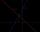Find the intersections of the function plot with coordinate axes: f (x): y = x + 3/5
6. A manA man spends 5/9 of his money on rent, and 5/16 of the remainder on electricity. If the final balance remaining is 550 find how much was spent on rent
7. Intercept with axisF(x)=log(x+4)-2, what is the x intercept
8. Last pageTwo consecutive sheets dropped out of the book. The sum of the numbers on the sides of the dropped sheets is 154. What is the number of the last page of the dropped sheets?
9. Twenty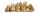Twenty rabbits are put in 4 cells so that there are different number of rabbits in each cell contains at least 3 rabbits. What is the largest possible number of rabbits in one cell
10. Mixture 2How many liters of water must be added to 7 liters of a 20% solution to obtain a 10% solution?
11. Taxi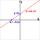A taxi ride costs \$3 plus \$2.50 per mile. Write and Graph an equation in two variables that represents the total cost of a taxi ride.
12. EquatiomSolve equation with negatives: X/(-5) + 2 = -9
13. Sand castle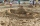Tim and Tom built a sand castle and embellished it with a flag. Half the pole with the flag plunged into the castle. The highest point of the pole was 80 cm above the ground, its lowest point 20 cm above the ground. How high was the sand castle?
14. Diofant equation250x + 120y = 5640
15. Midpoint 4If the midpoint of a segment is (6,3) and the other end point is (8,-4) what are thw coordinate of the other end?
16. Journey 5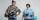A man has to do a journey of 84km in 3 hours. He travels the first 30km at 20km/hr. At what rate must he travel the remaining distance to complete his journey on time?
17. Find xSolve: if 2(x-1)=14, then x= (solve an equation with one unknown)
18. Value 4If 5/18=425 what is the value 13/18?
19. Two numbers 6Fill two natural numbers a, b: 7 + blank- blank = 5
20. FlourKim needs 3/4 cup of flour to make 12 cookies. How much flour would she need to make 60 cookies?

Do you have an interesting mathematical example that you can't solve it? Enter it, and we can try to solve it.

To this e-mail address, we will reply solution; solved examples are also published here. Please enter e-mail correctly and check whether you don't have a full mailbox.

Do you have a linear equation or system of equations and looking for its solution? Or do you have quadratic equation?